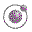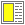Name binding, Recursion, Iteration, and Continuations - slide 16 : 42

Recursion
Recursive functions are indispensable for the processing of recursive data structures

Recursion is an algorithmic program solving idea that involves solution of subproblems of the same nature as the overall problem

• Given a problem P

• If P is trivial then solve it immediately

• If P is non-trivial, divide P into subproblems P1 ,..., Pn

• Observe if Pi (i=1..n) are of the same nature as P

• If so, use the overall problem solving idea on each of P1 ... Pn

• Combine solutions of the subproblems P1 ... Pn to a solution of the overall problem P